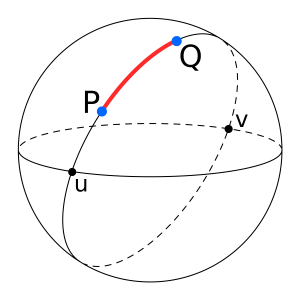Open In App

# Program for distance between two points on earth

Given latitude and longitude in degrees find the distance between two points on the earth.Image Source : Wikipedia
Examples:

```Input : Latitude 1: 53.32055555555556
Latitude 2: 53.31861111111111
Longitude 1: -1.7297222222222221
Longitude 2: -1.6997222222222223
Output: Distance is: 2.0043678382716137 Kilometers ```

Problem can be solved using Haversine formula:

The great circle distance or the orthodromic distance is the shortest distance between two points on a sphere (or the surface of Earth). In order to use this method, we need to have the co-ordinates of point A and point B.The great circle method is chosen over other methods.
First, convert the latitude and longitude values from decimal degrees to radians. For this divide the values of longitude and latitude of both the points by 180/pi. The value of pi is 22/7. The value of 180/pi is approximately 57.29577951. If we want to calculate the distance between two places in miles, use the value 3, 963, which is the radius of Earth. If we want to calculate the distance between two places in kilometers, use the value 6, 378.8, which is the radius of Earth.

Find the value of the latitude in radians:
Value of Latitude in Radians, lat = Latitude / (180/pi) OR
Value of Latitude in Radians, lat = Latitude / 57.29577951
Find the value of longitude in radians:
Value of Longitude in Radians, long = Longitude / (180/pi) OR
Value of Longitude in Radians, long = Longitude / 57.29577951

Get the co-ordinates of point A in terms of latitude and longitude. Use the above conversion method to convert the values of latitude and longitude in radians. I will call it as lat1 and long1. Do the same for the co-ordinates of Point B and get lat2 and long2.
Now, to get the distance between point A and point B use the following formula:

Distance, d = 3963.0 * arccos[(sin(lat1) * sin(lat2)) + cos(lat1) * cos(lat2) * cos(long2 – long1)]

The obtained distance, d, is in miles. If you want your value to be in units of kilometers, multiple d by 1.609344.
d in kilometers = 1.609344 * d in miles
Thus you can have the shortest distance between two places on Earth using the great circle distance approach.

## C++

 `// C++ program to calculate Distance``// Between Two Points on Earth``#include ``using` `namespace` `std;` `// Utility function for``// converting degrees to radians``long` `double` `toRadians(``const` `long` `double` `& degree)``{``    ``// cmath library in C++``    ``// defines the constant``    ``// M_PI as the value of``    ``// pi accurate to 1e-30``    ``long` `double` `one_deg = (M_PI) / 180;``    ``return` `(one_deg * degree);``}` `long` `double` `distance(``long` `double` `lat1, ``long` `double` `long1,``                     ``long` `double` `lat2, ``long` `double` `long2)``{``    ``// Convert the latitudes``    ``// and longitudes``    ``// from degree to radians.``    ``lat1 = toRadians(lat1);``    ``long1 = toRadians(long1);``    ``lat2 = toRadians(lat2);``    ``long2 = toRadians(long2);``    ` `    ``// Haversine Formula``    ``long` `double` `dlong = long2 - long1;``    ``long` `double` `dlat = lat2 - lat1;` `    ``long` `double` `ans = ``pow``(``sin``(dlat / 2), 2) +``                          ``cos``(lat1) * ``cos``(lat2) *``                          ``pow``(``sin``(dlong / 2), 2);` `    ``ans = 2 * ``asin``(``sqrt``(ans));` `    ``// Radius of Earth in``    ``// Kilometers, R = 6371``    ``// Use R = 3956 for miles``    ``long` `double` `R = 6371;``    ` `    ``// Calculate the result``    ``ans = ans * R;` `    ``return` `ans;``}` `// Driver Code``int` `main()``{``    ``long` `double` `lat1 = 53.32055555555556;``    ``long` `double` `long1 = -1.7297222222222221;``    ``long` `double` `lat2 = 53.31861111111111;``    ``long` `double` `long2 = -1.6997222222222223;``    ` `    ``// call the distance function``    ``cout << setprecision(15) << fixed;``    ``cout << distance(lat1, long1,``                     ``lat2, long2) << ``" K.M"``;` `    ``return` `0;``}` `// This code is contributed``// by Aayush Chaturvedi`

## Java

 `// Java program to calculate Distance Between``// Two Points on Earth``import` `java.util.*;``import` `java.lang.*;` `class` `GFG {` `    ``public` `static` `double` `distance(``double` `lat1,``                     ``double` `lat2, ``double` `lon1,``                                  ``double` `lon2)``    ``{` `        ``// The math module contains a function``        ``// named toRadians which converts from``        ``// degrees to radians.``        ``lon1 = Math.toRadians(lon1);``        ``lon2 = Math.toRadians(lon2);``        ``lat1 = Math.toRadians(lat1);``        ``lat2 = Math.toRadians(lat2);` `        ``// Haversine formula``        ``double` `dlon = lon2 - lon1;``        ``double` `dlat = lat2 - lat1;``        ``double` `a = Math.pow(Math.sin(dlat / ``2``), ``2``)``                 ``+ Math.cos(lat1) * Math.cos(lat2)``                 ``* Math.pow(Math.sin(dlon / ``2``),``2``);``            ` `        ``double` `c = ``2` `* Math.asin(Math.sqrt(a));` `        ``// Radius of earth in kilometers. Use 3956``        ``// for miles``        ``double` `r = ``6371``;` `        ``// calculate the result``        ``return``(c * r);``    ``}` `    ``// driver code``    ``public` `static` `void` `main(String[] args)``    ``{``        ``double` `lat1 = ``53.32055555555556``;``        ``double` `lat2 = ``53.31861111111111``;``        ``double` `lon1 = -``1.7297222222222221``;``        ``double` `lon2 = -``1.6997222222222223``;``        ``System.out.println(distance(lat1, lat2,``                           ``lon1, lon2) + ``" K.M"``);``    ``}``}` `// This code is contributed by Prasad Kshirsagar`

## Python3

 `# Python 3 program to calculate Distance Between Two Points on Earth``from` `math ``import` `radians, cos, sin, asin, sqrt``def` `distance(lat1, lat2, lon1, lon2):``    ` `    ``# The math module contains a function named``    ``# radians which converts from degrees to radians.``    ``lon1 ``=` `radians(lon1)``    ``lon2 ``=` `radians(lon2)``    ``lat1 ``=` `radians(lat1)``    ``lat2 ``=` `radians(lat2)``     ` `    ``# Haversine formula``    ``dlon ``=` `lon2 ``-` `lon1``    ``dlat ``=` `lat2 ``-` `lat1``    ``a ``=` `sin(dlat ``/` `2``)``*``*``2` `+` `cos(lat1) ``*` `cos(lat2) ``*` `sin(dlon ``/` `2``)``*``*``2` `    ``c ``=` `2` `*` `asin(sqrt(a))``   ` `    ``# Radius of earth in kilometers. Use 3956 for miles``    ``r ``=` `6371``     ` `    ``# calculate the result``    ``return``(c ``*` `r)``    ` `    ` `# driver code``lat1 ``=` `53.32055555555556``lat2 ``=` `53.31861111111111``lon1 ``=` `-``1.7297222222222221``lon2 ``=`  `-``1.6997222222222223``print``(distance(lat1, lat2, lon1, lon2), ``"K.M"``)`

## C#

 `// C# program to calculate``// Distance Between Two``// Points on Earth``using` `System;` `class` `GFG``{``    ``static` `double` `toRadians(``           ``double` `angleIn10thofaDegree)``    ``{``        ``// Angle in 10th``        ``// of a degree``        ``return` `(angleIn10thofaDegree * ``                       ``Math.PI) / 180;``    ``}``    ``static` `double` `distance(``double` `lat1,``                           ``double` `lat2,``                           ``double` `lon1,``                           ``double` `lon2)``    ``{` `        ``// The math module contains``        ``// a function named toRadians``        ``// which converts from degrees``        ``// to radians.``        ``lon1 = toRadians(lon1);``        ``lon2 = toRadians(lon2);``        ``lat1 = toRadians(lat1);``        ``lat2 = toRadians(lat2);` `        ``// Haversine formula``        ``double` `dlon = lon2 - lon1;``        ``double` `dlat = lat2 - lat1;``        ``double` `a = Math.Pow(Math.Sin(dlat / 2), 2) +``                   ``Math.Cos(lat1) * Math.Cos(lat2) *``                   ``Math.Pow(Math.Sin(dlon / 2),2);``            ` `        ``double` `c = 2 * Math.Asin(Math.Sqrt(a));` `        ``// Radius of earth in``        ``// kilometers. Use 3956``        ``// for miles``        ``double` `r = 6371;` `        ``// calculate the result``        ``return` `(c * r);``    ``}` `    ``// Driver code``    ``static` `void` `Main()``    ``{``        ``double` `lat1 = 53.32055555555556;``        ``double` `lat2 = 53.31861111111111;``        ``double` `lon1 = -1.7297222222222221;``        ``double` `lon2 = -1.6997222222222223;``        ``Console.WriteLine(distance(lat1, lat2,``                          ``lon1, lon2) + ``" K.M"``);``    ``}``}` `// This code is contributed by``// Manish Shaw(manishshaw1)`

## PHP

 ``

## Javascript

 ``

Output

`2.004367838271690 K.M`

Time Complexity: O(logn) as inbuilt sqrt function has been used
Auxiliary Space: O(1)

Please suggest if someone has a better solution which is more efficient in terms of space and time.